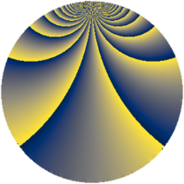# Properties

 Label 1110.2.brLevel $1110$ Weight $2$ Character orbit 1110.br Rep. character $\chi_{1110}(151,\cdot)$ Character field $\Q(\zeta_{18})$ Dimension $144$ Sturm bound $456$

# Related objects

## Defining parameters

 Level: $$N$$ $$=$$ $$1110 = 2 \cdot 3 \cdot 5 \cdot 37$$ Weight: $$k$$ $$=$$ $$2$$ Character orbit: $$[\chi]$$ $$=$$ 1110.br (of order $$18$$ and degree $$6$$) Character conductor: $$\operatorname{cond}(\chi)$$ $$=$$ $$37$$ Character field: $$\Q(\zeta_{18})$$ Sturm bound: $$456$$

## Dimensions

The following table gives the dimensions of various subspaces of $$M_{2}(1110, [\chi])$$.

Total New Old
Modular forms 1416 144 1272
Cusp forms 1320 144 1176
Eisenstein series 96 0 96

## Trace form

 $$144q + O(q^{10})$$ $$144q + 12q^{10} - 36q^{14} - 60q^{19} + 72q^{29} + 48q^{33} + 36q^{34} - 24q^{35} - 144q^{36} + 48q^{37} + 48q^{38} + 24q^{39} + 48q^{42} + 12q^{44} + 12q^{46} + 24q^{47} + 12q^{55} - 48q^{62} + 72q^{64} - 12q^{65} - 24q^{71} + 48q^{73} + 24q^{74} - 60q^{76} + 168q^{77} - 24q^{79} - 24q^{83} - 24q^{85} - 72q^{86} - 24q^{87} - 36q^{89} + 60q^{91} + 24q^{92} - 72q^{93} + 12q^{94} - 72q^{97} - 96q^{98} - 12q^{99} + O(q^{100})$$

## Decomposition of $$S_{2}^{\mathrm{new}}(1110, [\chi])$$ into newform subspaces

The newforms in this space have not yet been added to the LMFDB.

## Decomposition of $$S_{2}^{\mathrm{old}}(1110, [\chi])$$ into lower level spaces

$$S_{2}^{\mathrm{old}}(1110, [\chi]) \cong$$ $$S_{2}^{\mathrm{new}}(37, [\chi])$$$$^{\oplus 8}$$$$\oplus$$$$S_{2}^{\mathrm{new}}(74, [\chi])$$$$^{\oplus 4}$$$$\oplus$$$$S_{2}^{\mathrm{new}}(111, [\chi])$$$$^{\oplus 4}$$$$\oplus$$$$S_{2}^{\mathrm{new}}(185, [\chi])$$$$^{\oplus 4}$$$$\oplus$$$$S_{2}^{\mathrm{new}}(222, [\chi])$$$$^{\oplus 2}$$$$\oplus$$$$S_{2}^{\mathrm{new}}(370, [\chi])$$$$^{\oplus 2}$$$$\oplus$$$$S_{2}^{\mathrm{new}}(555, [\chi])$$$$^{\oplus 2}$$